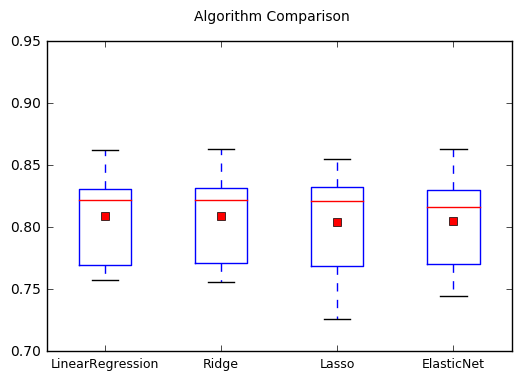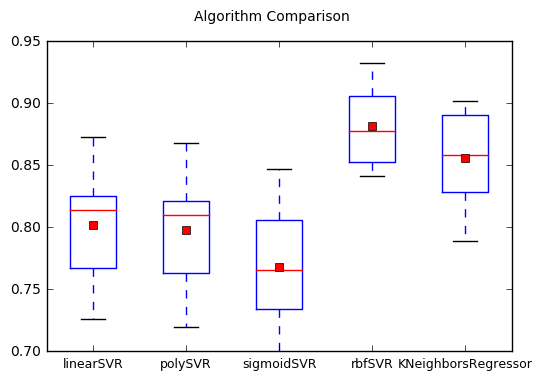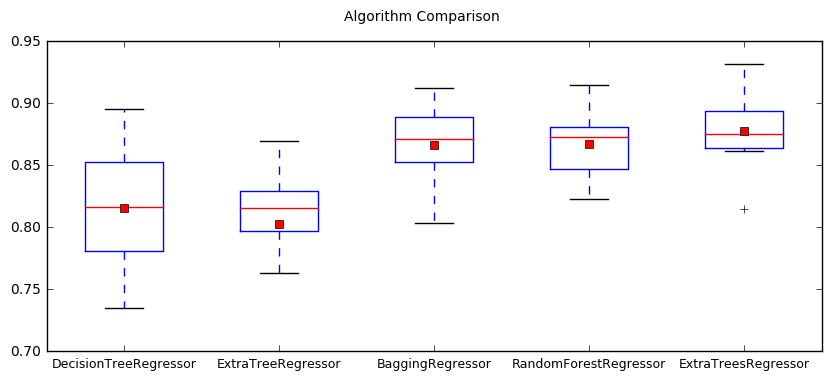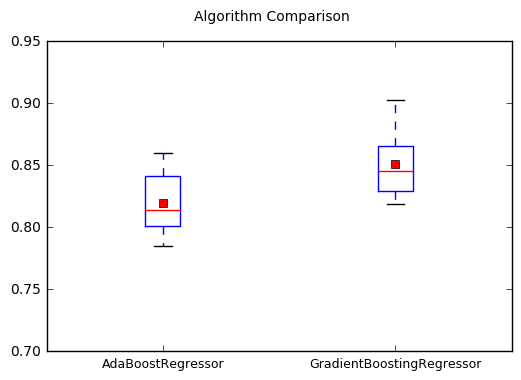# Optimization of ML Regression Models¶

In this project, we will compare the performance of different machine learning regression models on the Auto-MPG data set. In addition, we will look into how to optimize each model for best performance.

The PipeLine and GridSearch tools from the Scikit-Learn library will be utilized.

The following regression models will be studied:

• Linear Models: LinearRegression, Ridge, Lasso and ElasticNet
• K-nearest Neighbor Regression: KNeighborsRegressor
• Support Vector Regression: SVR with linear, poly, sigmoid and rbf kernels
• Decision Tree Regression: DecisionTreeRegressor and ExtraTreeRegressor
• Bagging Algorithms: BaggingRegressor, RandomForestRegressor and ExtraTreesRegressor
• Boosting Algorithms: AdaBoostRegressor and GradientBoostingRegressor

For this project we will use the Auto MPG data set. Please follow the instructions in the post Working with the Auto MPG Data Set to get yourself familiar with the dataset, prepare the data for analysis and generate the auto-mpg.csv that will be used in this project.

Sections 1 in the previous post titled Impact of Scaling on Machine Learning Algorithms go over the scaling differences in the data in detail.

Below, the code that separates the data into inputs, X and output, y is provided.

In :
import pandas as pd
import numpy as np

filename = "auto-mpg.csv"
output = df.iloc[:,0]
features = df.iloc[:, 1:8]
X = features.values
y = output.values

Out:
mpg cylinders displacement horsepower weight acceleration year origin name
0 18.0 8 307.0 130.0 3504.0 12.0 70 1 chevrolet chevelle malibu
1 15.0 8 350.0 165.0 3693.0 11.5 70 1 buick skylark 320
2 18.0 8 318.0 150.0 3436.0 11.0 70 1 plymouth satellite

The y variable holds the mpg column data and the X variable holds the data in the cylinders, displacement, horsepower, weight, acceleration, year and origin columns (the name column is not used in this project).

## 2. Test and Display Functions¶

The following funtions will be used in the remaining parts of this post.

In :
def get_modelname(model):
name = str(model).partition('(')
if name=='SVR':
name = model.get_params()['kernel'] + name
return(name)


get_modelname extracts the name of the model from the model function call. For support vector regression, the kernel name is also prepended to the model name.

In :
def evalModels(models, Xw, y, boxPlotOn=True):
from sklearn.preprocessing import StandardScaler # Standardize data (0 mean, 1 stdev)
from sklearn.model_selection import KFold
from sklearn.model_selection import cross_val_score
from sklearn.pipeline import Pipeline, make_pipeline

scoring = 'r2'  #'neg_mean_squared_error'
modelnames = []
results = []
for model in models:
pipe = make_pipeline( StandardScaler(), model )
kfold = KFold(n_splits=8, random_state=6, shuffle=True)
cv_results = cross_val_score(pipe, Xw, y, cv=kfold, scoring=scoring)
modelname = get_modelname(model)
print "%s: %.3f %.3f" %(modelname, cv_results.mean(), cv_results.std())
modelnames.append(modelname)
results.append(cv_results)

if boxPlotOn:
import matplotlib.pyplot as plt
# boxplot algorithm comparison
fig = plt.figure()
fig.suptitle('Algorithm Comparison')
plt.boxplot(results, showmeans=True)
ax.set_xticklabels(modelnames, fontsize=9)
plt.ylim(0.7, 0.95)
plt.show()

return(results)


The evalModels function includes the following:

• A pipeline that includes standardization of the input vectors (so that variance is 1 and mean is zero) and application of the ML model.
• 8-split, shuffled cross-validation
• Box plotting all the performance results

## 3. Pipelining transformers and predictors¶

It is important to extract tranformation parameters (such as scaling) from only the training data and keep the testing data untouched in this regard. The most convenient way to accomplish this by using the pipelining feature in scikit-learn.

The following example from the scikit-learn website shows how to achieve this without pipelining:

In [ ]:
from sklearn import preprocessing

X_train, X_test, y_train, y_test = train_test_split(
iris.data, iris.target, test_size=0.4, random_state=0)
scaler = preprocessing.StandardScaler().fit(X_train)
X_train_transformed = scaler.transform(X_train)
clf = svm.SVC(C=1).fit(X_train_transformed, y_train)
X_test_transformed = scaler.transform(X_test)
clf.score(X_test_transformed, y_test)


The following sample code using a pipeline, however, is much simpler, allows treating the pipeline as a model so that multi-split cross validation can be applied easily:

In [ ]:
from sklearn.pipeline import make_pipeline
clf = make_pipeline(preprocessing.StandardScaler(), svm.SVC(C=1))
cross_val_score(clf, iris.data, iris.target, cv=cv)


In this project, all performance simulations are run using a pipeline formed by calling the function evalModels above.

## 4. Comparison of ML regression models¶

In this section we run all regression models using 8-split cross-validation and compare the R² results.

All regressors have been manually optimized. In other words, a manual search has been conducted in the parameter space of each regressor for the best performing parameter set which was then used to call each model. The default setting is kept for those parameters that did not make any noticeable difference.

All input data has been standardized on the training data set.

### 4.1 Linear Regression¶

In :
from sklearn.linear_model import LinearRegression, Ridge, Lasso, ElasticNet

# create and evaluate pipeline
models = []
models.append( LinearRegression() )
models.append( Ridge(alpha=1.0) )
models.append( Lasso(alpha=0.5) )
models.append( ElasticNet(alpha=0.1, l1_ratio=0.5) )

Xw = X
results = evalModels(models, Xw, y, boxPlotOn=True)

LinearRegression: 0.809 0.036
Ridge: 0.809 0.037
Lasso: 0.804 0.042
ElasticNet: 0.805 0.038All linear regression models perform similarly. The linear models with regularization most likely act like a standard linear regressor since the data is standardized. Top performing score is R²=0.81 with std 0.36 achieved by standard linear regression.

### 4.2 K-Neigbors and Support Vector Regression¶

In :
from sklearn.svm import SVR
from sklearn.neighbors import KNeighborsRegressor

# create and evaluate pipeline
models = []
models.append( SVR(kernel='linear', C=10, epsilon=0.3, shrinking=False) )
models.append( SVR(kernel='poly', C=4, epsilon=0.1, degree=1) )
models.append( SVR(kernel='sigmoid', C=0.2) )
models.append( SVR(kernel='rbf', C=20, epsilon=1.5) )
models.append( KNeighborsRegressor(weights='distance', p=2) )

Xw = X
results = evalModels(models, Xw, y, boxPlotOn=True)

linearSVR: 0.802 0.044
polySVR: 0.798 0.044
sigmoidSVR: 0.768 0.052
rbfSVR: 0.882 0.034
KNeighborsRegressor: 0.856 0.037Both K-nearest neighbor and support vector machine regressors are distance-based predictors.

The support vector regressor with a linear kernel, linearSVR, somewhat mimics the linear regression results above. SVR with a polynomial kernel, polySVR, converges to linearSVR in performance as degree of the polynomial kernel is reduced to 1. The sigmoidSVR using a sigmoid kernel is the most inferior of the SVR models.

The SVR model with a radial-basis-function, rbfSVR, produces the best performance with R²=0.88 and std 0.34. This is the best result achieved in this study.

The K-neighbors regressor performance is also very strong.

### 4.3 Decision Tree and Bagging regression algorithms¶

In :
from sklearn.tree import DecisionTreeRegressor
from sklearn.tree import ExtraTreeRegressor
from sklearn.ensemble import BaggingRegressor
from sklearn.ensemble import RandomForestRegressor
from sklearn.ensemble import ExtraTreesRegressor

# create and evaluate pipeline
models = []
models.append( DecisionTreeRegressor(max_leaf_nodes=9, min_samples_leaf=18) )
models.append( ExtraTreeRegressor(criterion='mae', max_leaf_nodes=50, min_samples_leaf=4, min_samples_split=6) )
cart = DecisionTreeRegressor()
models.append( BaggingRegressor(base_estimator=cart, random_state=7, n_estimators=300, max_features=6) )
models.append( RandomForestRegressor(n_estimators=100, max_features=4, criterion='mae', max_leaf_nodes=50, min_samples_split=4) )
models.append( ExtraTreesRegressor(n_estimators=100, max_features=4, max_depth=1000, min_samples_split=6) )

Xw = X
plt.rcParams["figure.figsize"] = [10, 4]
results = evalModels(models, Xw, y, boxPlotOn=True)
plt.rcParams["figure.figsize"] = [6, 4]

DecisionTreeRegressor: 0.816 0.052
ExtraTreeRegressor: 0.803 0.051
BaggingRegressor: 0.867 0.035
RandomForestRegressor: 0.867 0.030
ExtraTreesRegressor: 0.878 0.033In this plot we are looking at the perfomance of decision tree variants of regression models. Albeit with a higher std, the basic decision tree regressor, DecisionTreeRegressor, performs on average slightly better than linear regression models and its sibling, the ExtraTreeRegressor.

The three bagging algorithms on the right employ 100-300 trees and show a strong performance with the ExtraTrees bagging algorithm almost matching the top performance of rbfSVR arriving at R²=0.88 and std 0.33.

### 4.4 Boosting methods¶

In :
from sklearn.ensemble import AdaBoostRegressor

# create and evaluate pipeline
models = []
models.append( GradientBoostingRegressor(n_estimators=80, criterion='mae', max_features=5, min_samples_split=15, random_state=7) )

Xw = X
results = evalModels(models, Xw, y, boxPlotOn=True)

AdaBoostRegressor: 0.820 0.026The boosting methods are also decision-tree based as the models covered in the previous section although the optimum number of trees were found to be much lower than that of the bagging methods: 10 for AdaBoost and 80 for GradientBoosting. Although their average peformance is somewhat lower than the bagging methods, the boosting methods has the lowest std in performance so far: 0.26 for AdaBoost.

### 4.5 Comparison Summary¶

Below is the list of ML methods producing an average R² value larger than 0.85 starting with the highest score:

• SVR with rbf kernel : 0.882 with std of 0.034
• ExtraTreesRegressor : 0.878 with std of 0.033
• RandomForestRegressor : 0.867 with std of 0.030
• BaggingRegressor : 0.867 with std of 0.035
• KNeighborsRegressor : 0.856 with std of 0.037
• GradientBoostingRegressor: 0.851 with std of 0.027

## 5. Algorithm Tuning¶

### 5.1 Grid Search Parameter Tuning¶

In :
# Standardize data (0 mean, 1 stdev)
from sklearn.preprocessing import StandardScaler
scaler = StandardScaler().fit(X)
standardizedX = scaler.transform(X)
Xw = standardizedX
from sklearn.model_selection import KFold

# Grid Search for Algorithm Tuning
from sklearn.linear_model import Ridge
from sklearn.model_selection import GridSearchCV

alphas = np.array([1,0.5,0.2,0.1,0.01,0.001,0.0001,0])
param_grid = dict(alpha=alphas)
model = Ridge()
kfold = KFold(n_splits=8, random_state=6, shuffle=True)

grid = GridSearchCV(estimator=model, param_grid=param_grid, cv=kfold)
grid.fit(Xw, y)
print(grid.best_score_)
print(grid.best_estimator_.alpha)
#print grid.cv_results_

0.809570605568
1.0


### 5.2 Random Search Parameter Tuning¶

In :
Xw = standardizedX

# Randomized for Algorithm Tuning
from scipy.stats import uniform
from sklearn.linear_model import Ridge
from sklearn.model_selection import RandomizedSearchCV

param_grid = {'alpha': uniform()}
seed=6
model = Ridge()
kfold = KFold(n_splits=8, random_state=seed, shuffle=True)

rsearch = RandomizedSearchCV(estimator=model, param_distributions=param_grid, n_iter=100, cv=kfold, random_state=seed)
rsearch.fit(Xw, y)
print(rsearch.best_score_)
print(rsearch.best_estimator_.alpha)

0.809570412842
0.994207438422

In [ ]: